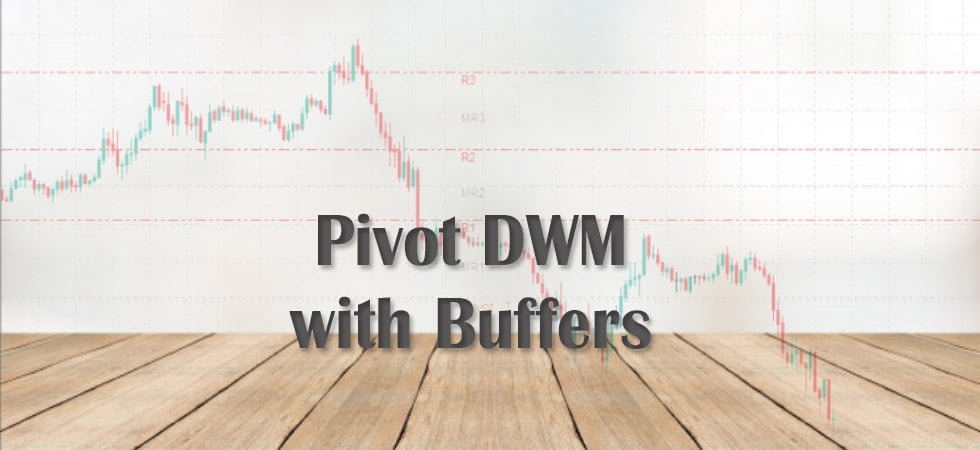# MQ4: Pivot DWM Get Buffer Values from Objects

## Pivot DWM:

This is an amazing indicator which calculates Pivot Points and draws them on the screen. Unfortunately, it does not have buffers. This post will show you how to get the Pivot Points: R1, R2, R3, P, S1, S2, S3 into buffers.## Wrapper Indicator:

You’ll need to create a wrapper indicator which will get Object Values from Chart once for each bar and save them into Buffers.

• ### Buffer Arrays:

`#property indicator_buffers 7`
```double R1[];
double R2[];
double R3[];

double P[];

double S1[];
double S2[];
double S3[];```
• ### Init

```IndicatorBuffers(7);
SetIndexBuffer(0, R3);
SetIndexLabel(0, "R3");

SetIndexBuffer(1, R2);
SetIndexLabel(1, "R2");

SetIndexBuffer(2, R1);
SetIndexLabel(2, "R1");

SetIndexBuffer(3, P);
SetIndexLabel(3, "P");

SetIndexBuffer(4, S1);
SetIndexLabel(4, "S1");

SetIndexBuffer(5, S2);
SetIndexLabel(5, "S2");

SetIndexBuffer(6, S3);
SetIndexLabel(6, "S3");```

These Pivot Points are from Top to Bottom. R3 (Resistance 3) is top-most and S3 (Support 3) is the bottom-most.

• ### Calculate only for new bars

```int limit;
int counted_bars=IndicatorCounted();

if(counted_bars<0) return(-1);

if(counted_bars>0) counted_bars--;
limit=Bars-counted_bars;```

This code will make sure that values for only new bars are calculated

• ### Calculate only once per bar

```datetime lastBarOpenTime;

//+------------------------------------------------------------------+
// Check if Current Tick is in NewBar
//+------------------------------------------------------------------+
bool IsNewBar()
{
datetime thisBarOpenTime = Time;
if(thisBarOpenTime != lastBarOpenTime)
{
lastBarOpenTime = thisBarOpenTime;
return (true);
}
else {
return (false);
}
}```

This function checks if a bar is a New Bar.

• ### Get Values from Objects

```iCustom(NULL, 0, "Pivot DWM", 0,0);

for(int j=0;j<obj_total;j++)
{
name=ObjectName(j);

// Get Pivot Line Names and Values (Price)
if(StringFind(name, "line", 0) > -1) {

string pname = name;
StringReplace(pname, " line", "");
double pvalue = NormalizeDouble(ObjectGet(name, OBJPROP_PRICE1), Digits);

if(pname == "R1") {
R1[i] = pvalue;
} else if(pname == "R2") {
R2[i] = pvalue;
} else if(pname == "R3") {
R3[i] = pvalue;
} else if(pname == "P") {
P[i] = pvalue;
} else if(pname == "S1") {
S1[i] = pvalue;
} else if(pname == "S2") {
S2[i] = pvalue;
} else if(pname == "S3") {
S3[i] = pvalue;
}
}
}```

Copy all files into your MQL4 Indicators folder.

## Some Remarks:

• There might be a problem if you have another indicator on Chart which draws objects with name ” line” in it.
• Also the buffer values for previous bars before adding this indicator to screen will not be calculated
• To calculate mid-levels like MR3, you can always do R3-R2 and so on. Possible mid-levels: MR3, MR2, MR1, MS1, MS2, MS3.

There is another similar post for calculating Buffer values in another indicator. It has code to calculate arrows as well.